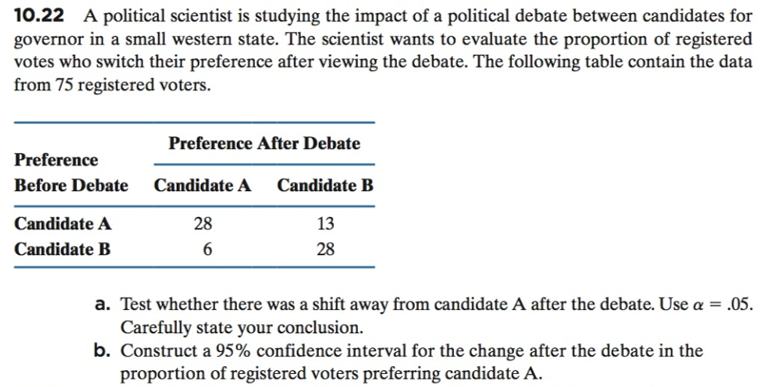# 10.22 A political scientist is studying the impact of a political debate between candidates forgovernor in a small western state. The scientist wants to evaluate the proportion of registeredvotes who switch their preference after viewing the debate. The following table contain the datafrom 75 registered voters.Preference After DebatePreferenceBefore DebateCandidateACandidate BCandidate ACandidate B132828a. Test whether there was a shift away from candidate A after the debate. Use a .05Carefully state your conclusion.b. Construct a 95% confidence interval for the change after the debate in theproportion of registered voters preferring candidate A

Question
100 viewshelp_outlineImage Transcriptionclose10.22 A political scientist is studying the impact of a political debate between candidates for governor in a small western state. The scientist wants to evaluate the proportion of registered votes who switch their preference after viewing the debate. The following table contain the data from 75 registered voters. Preference After Debate Preference Before Debate CandidateA Candidate B Candidate A Candidate B 13 28 28 a. Test whether there was a shift away from candidate A after the debate. Use a .05 Carefully state your conclusion. b. Construct a 95% confidence interval for the change after the debate in the proportion of registered voters preferring candidate A fullscreen
check_circle

Step 1

Part (a): Test:

Let us first test whether “Preference before debate” and “Preference after debate” debate are independent or not, using a Chi-square test of Independence. If the two preferences are found not to be independent, then, by observing the change in the proportions, it can be decided whether there was a shift away from candidate A after the debate.

Hypotheses:

The aim is to check whether the illiteracy is higher in men than in women in Qatar.

The null hypothesis is:

H : The two preferences are independent.

The alternative hypothesis is:

H : The two preferences are not independent.

Calculation steps:

The calculations have been done in EXCEL.

Denote oij as the observed frequency for ith row and jth column (i =1, 2; j =1, 2) and eij as the expected frequency for ith row and jth column.

Table 1 provides the data values, oij.

Table 2 calculates the row proportions: (cell value)/(row total) = oij / oi∙ so that, the first row proportion will be 28/41 ≈ 0.682. Similarly, the others can be calculated.

Table 3 calculates the expected frequencies under the independence assumption. If independent, eij = (oi∙) ∙ (o∙j) / N. [o∙j = column total of jth column, N = grand total of all observations = 75]. So, the first cell expected frequency will be e11 = (41) ∙ (34) / 75 = 18.6. Similarly, the others can be calculated.

Test statistic:

The formula for the test statistic is χ2 = ∑ [(oijeij)2 / eij], summed over all i and j.

Table 4 calculates [(oijeij)2 / eij] for each (i, j). So, the value in the first cell will be (28 – 18.6)2 / 18.6 ≈ 4.751.

The test statistic value can be calculated by adding all these cell values.

The degrees of freedom is (2 – 1) ∙ (2 – 1) = 1.

Step 2

Test statistic value:

The test statistic value is: 4.751 + 3.945 + 5.738 + 4.751 = 19.183.

Critical value:

The level of significance is α = 0.05. Therefore, the critical value will be χ20.05.

From EXCEL formula “=CHISQ.INV.RT(0.05, 1)”, the critical value χ20.05 = 3.84.

Decision rule:

• If the test statistic is greater that the critical value (), reject the null hypothesis.
• Otherwise fail to reject the null hypothesis.

Conclusion:

Here, the test statistic, χ2 = 19.183 is greater than the critical value, χ20.05 = 3.84.

Therefore, by the decision rule, reject the null hypothesis.

...

### Want to see the full answer?

See Solution

#### Want to see this answer and more?

Solutions are written by subject experts who are available 24/7. Questions are typically answered within 1 hour.*

See Solution
*Response times may vary by subject and question.
Tagged in